# 数据挖掘入门系列教程（六）之数据集特征选择

2019/04/10 10:10

[TOC]

## 数据挖掘入门系列教程（六）之数据集特征选择

• 数据集太大，这个太耗费人力
• 如果某个关键的特征取值很相近（或者相同）怎么办

### 简介

• 数值型：数值特征，比如说Iris的花瓣长度是多少等等
• 类别型：类别特征（也可以称之为名义特征）比如说西瓜的颜色是浅绿色还是深绿色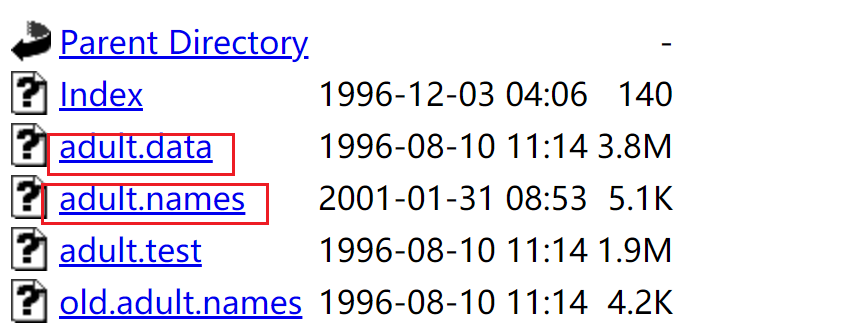adult.data中的部分数据如下，每一行代表的是一个人的数据，每一列表示的特征属性值（至于特征是什么，这个在adult.name文件中有介绍）：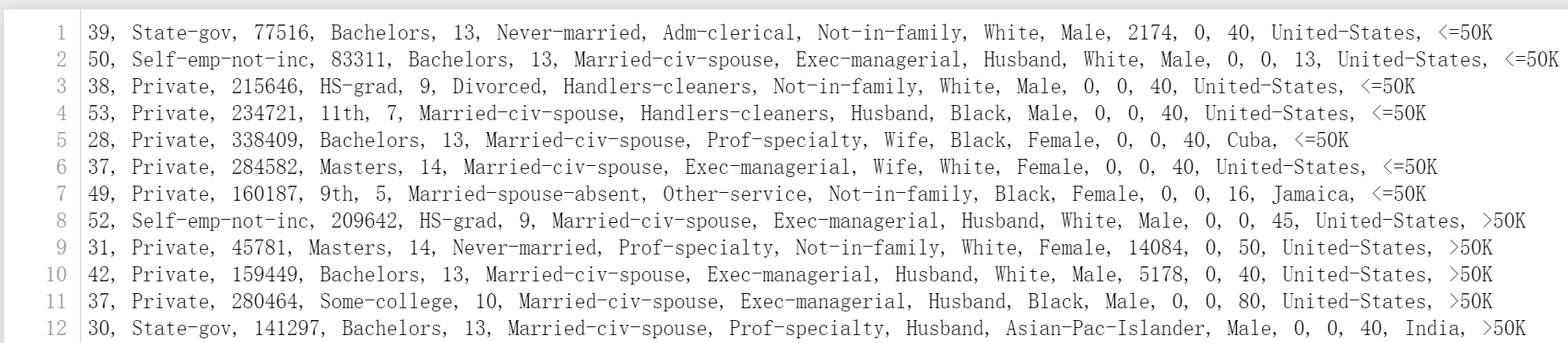🆗，现在我们就可以使用python来加载数据集了。使用pandas，这个前面已经介绍了。

import pandas as pd


names表示的就是每一个特征的名字。adult_data的数据如下。特征代表什么意思，基本上通过特征名就可以理解了。如果不理解的可以看adult.name文件。最后一个特征为money，他是分类结果，含义是他每年的收入是否大于50K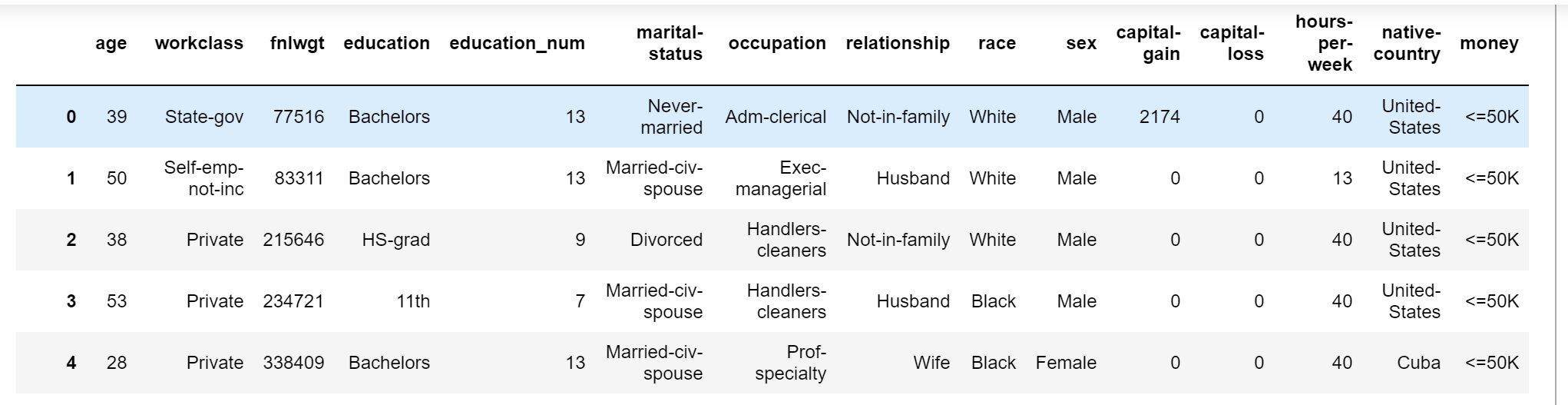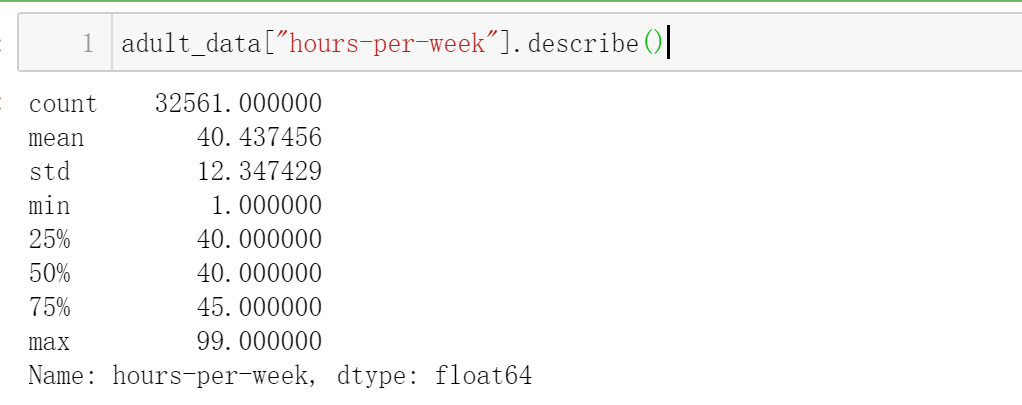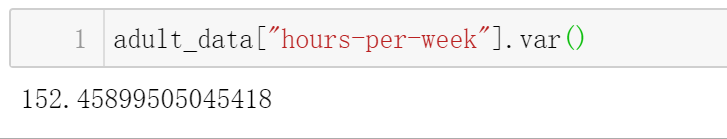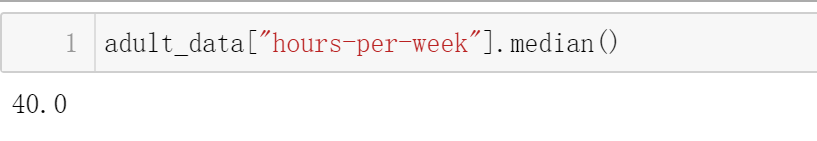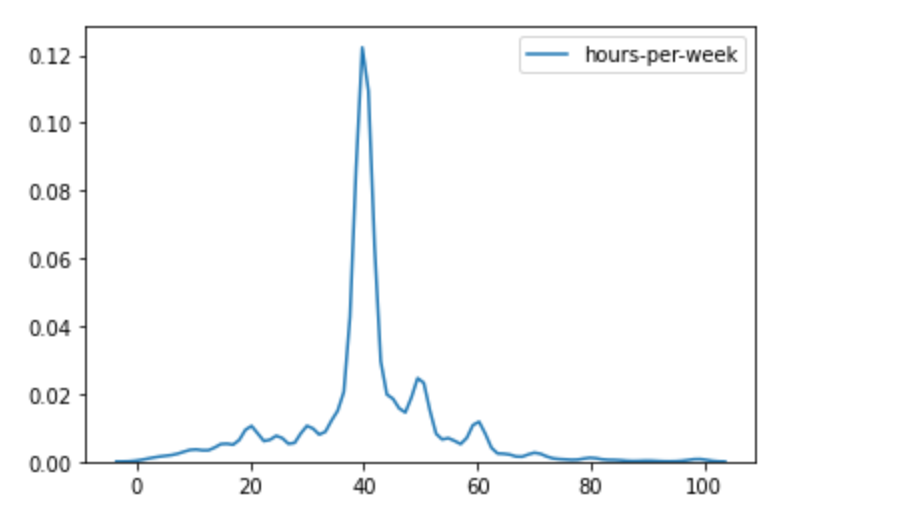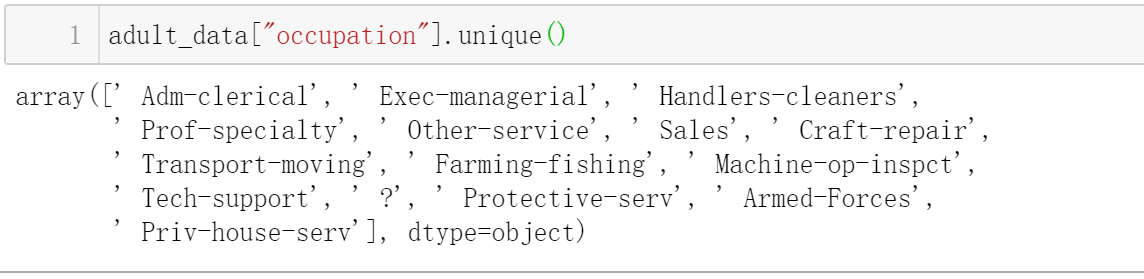?代表数据缺失。

### 特征选择

• 降低复杂度：特征越小，我们耗费的计算时间也就越少。
• 降低噪音：比如说西瓜分类中，西瓜的id就毫无作用。
• 增加模型的可读性。

#### 方差

import numpy as np
X = np.arange(30).reshape((10, 3))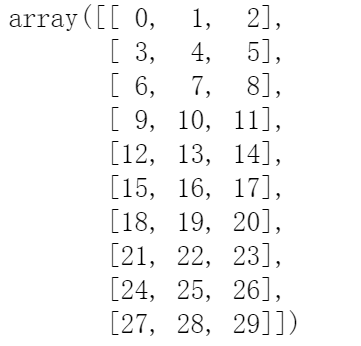X[:,1] = 1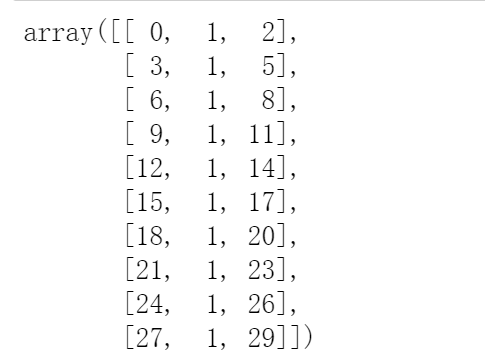from sklearn.feature_selection import VarianceThreshold
# threshold代表的就是阈值，默认是0.0
vt = VarianceThreshold(threshold=0.0)
Xt = vt.fit_transform(X)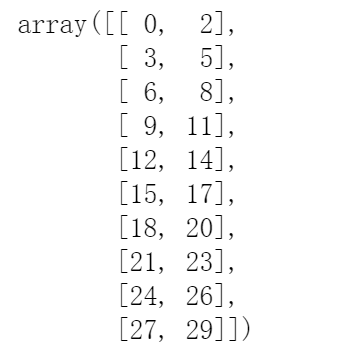VarianceThreshold有两个重要的函数：fittransform，这些说明官网都有，这里稍微的啰嗦以下。fit函数是去计算array的方差，而transform函数就是去转换array数组，将反差小于阈值的去除。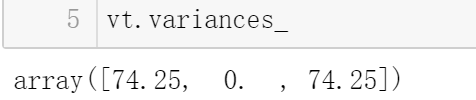X = adult_data[["age","education_num","capital-gain","capital-loss","hours-per-week"]]
from sklearn.tree import DecisionTreeClassifier
from sklearn.model_selection import cross_val_score
dtc = DecisionTreeClassifier(random_state=14)
score = cross_val_score(dtc, X, Y, scoring='accuracy')
score.mean()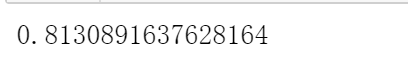from sklearn.feature_selection import VarianceThreshold
vt = VarianceThreshold(threshold=200)
Xt = vt.fit_transform(X)

# 构建决策树
from sklearn.tree import DecisionTreeClassifier
from sklearn.model_selection import cross_val_score
dtc = DecisionTreeClassifier(random_state=14)
score = cross_val_score(dtc, Xt, Y, scoring='accuracy')
score.mean()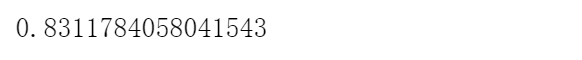### 选择最佳特征

• SelectKBest：选择k个最佳的特征。
• SelectPercentile：选择最佳的前r%g个特征。

#### 卡方验证$X^2$（Chi-Square Test）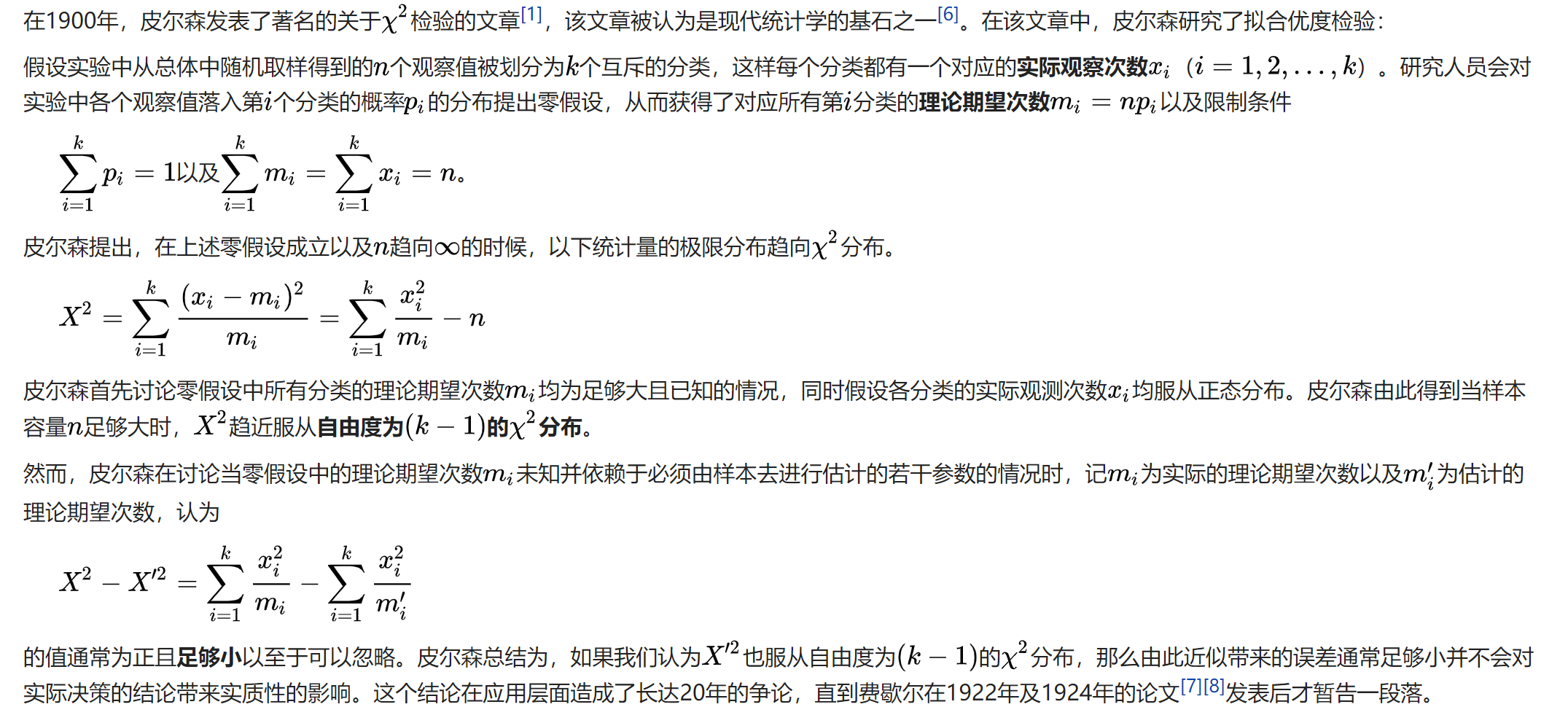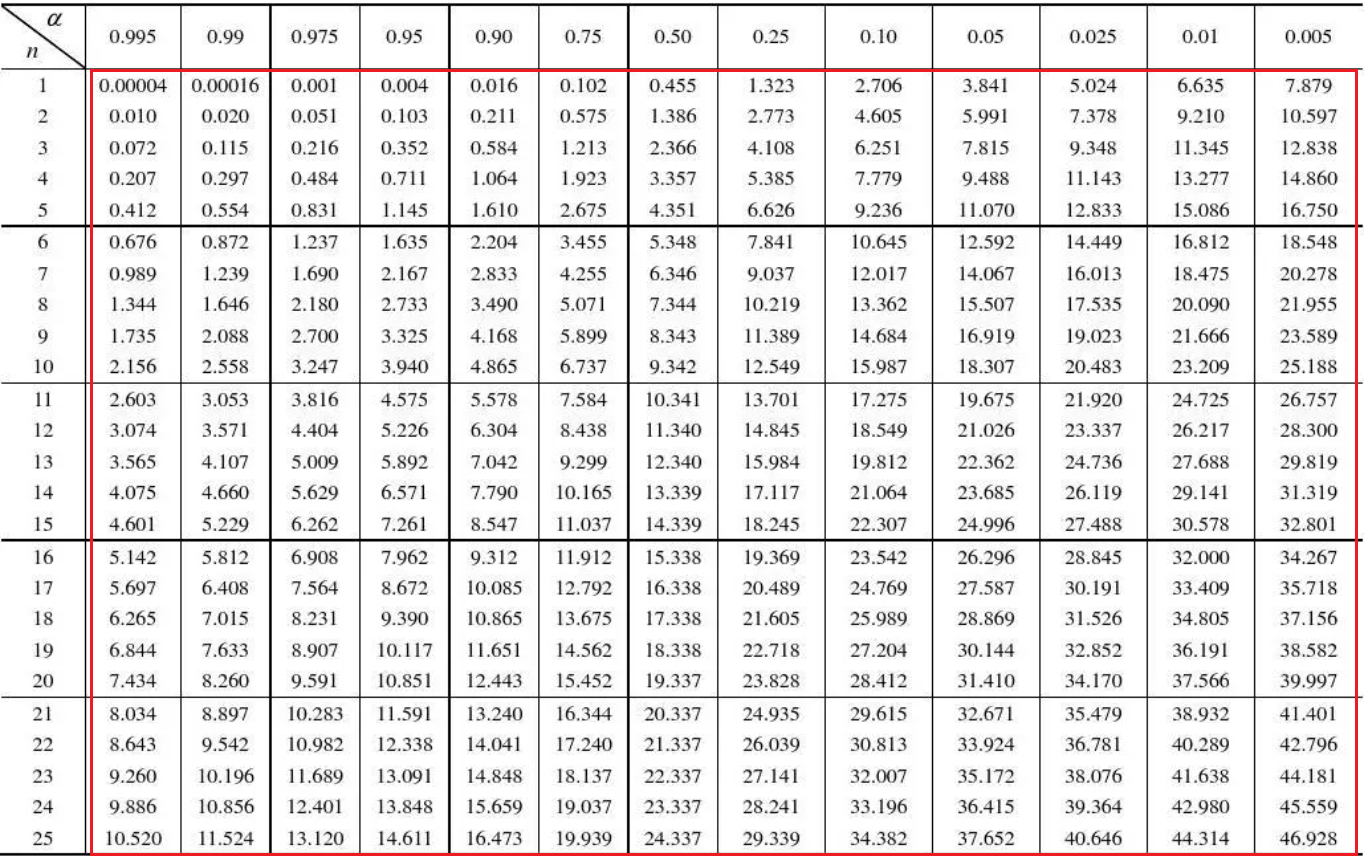X = adult_data[["age","education_num","capital-gain","capital-loss","hours-per-week"]].values

from sklearn.feature_selection import SelectKBest
# 导入卡方验证
from sklearn.feature_selection import chi2
# 选取前3个最好的节点
transformer = SelectKBest(score_func=chi2,k=3)
X_chi2 = transformer.fit_transform(X,Y)
transformer.scores_from sklearn.tree import DecisionTreeClassifier
from sklearn.model_selection import cross_val_score
dtc = DecisionTreeClassifier(random_state=14)
score = cross_val_score(dtc, X_chi2, Y, scoring='accuracy')
score.mean()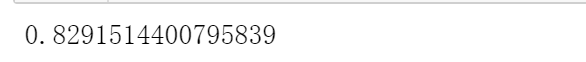emm，比方差的方法稍微差了一点。

#### 皮尔逊相关系数（Pearson Correlation Coefficient）

• 当相关系数为0时，X和Y两变量无关系。
• 当X的值增大（减小），Y值增大（减小），两个变量为正相关，相关系数在0.00与1.00之间。
• 当X的值增大（减小），Y值减小（增大），两个变量为负相关，相关系数在-1.00与0.00之间。

from scipy.stats import pearsonr
def pearsonr_fit(x,y):
scores=[]
p_values = []
for column in range(X.shape):
# cur_p表示的双侧p值，x[:,column]表示的是X中的某一列
# 然后计算X中的某一列与y之间得到关系，返回相关系数和p_value
cur_score,cur_p = pearsonr(x[:,column],y)
# 因为相关系数可能为负数，所以取绝对值
scores.append(abs(cur_score))
p_values.append(cur_p)
return (np.array(scores),np.array(p_values))


import numpy as np
# 这里不使用">50K"等字符串是因为pearsonr不接受字符串数据。
Y = adult_data["money"] == " >50K"
transformer = SelectKBest(score_func=pearsonr_fit,k=3)
X_pearsonr = transformer.fit_transform(X,Y)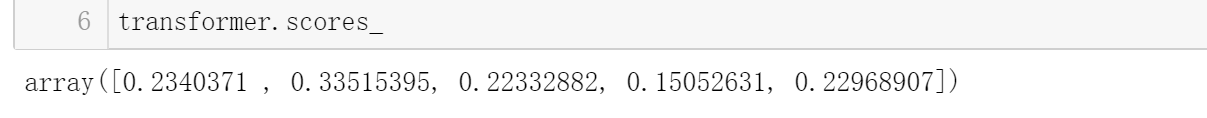from sklearn.tree import DecisionTreeClassifier
from sklearn.model_selection import cross_val_score
dtc = DecisionTreeClassifier(random_state=14)
score = cross_val_score(dtc, X_pearsonr, Y, scoring='accuracy')
score.mean()emm，更低了。

#### PCA 主成分分析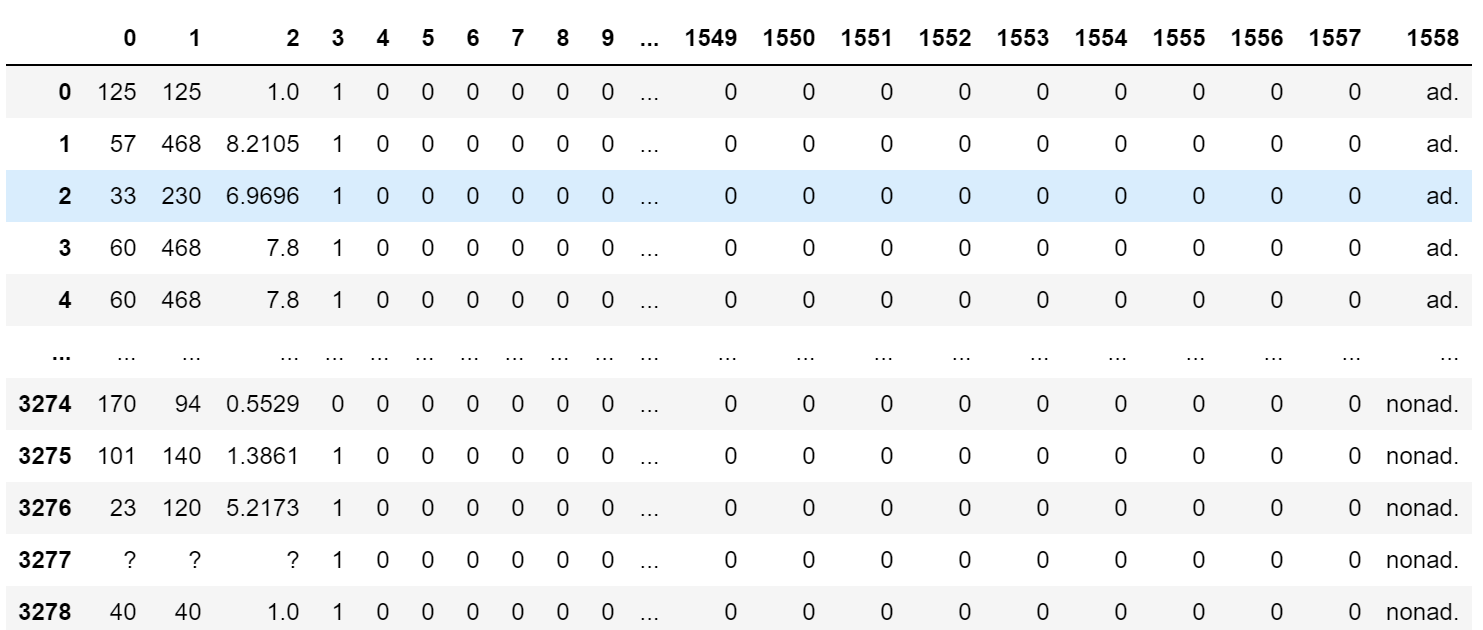import pandas as pd
import numpy as np
from collections import defaultdict

def convert_number(x):
try:
return float(x)
except ValueError:
return np.nan

converters = defaultdict()
for i in range(1559 -1):
converters[i] = convert_number
converters = lambda x:1 if x.strip() == "ad." else 0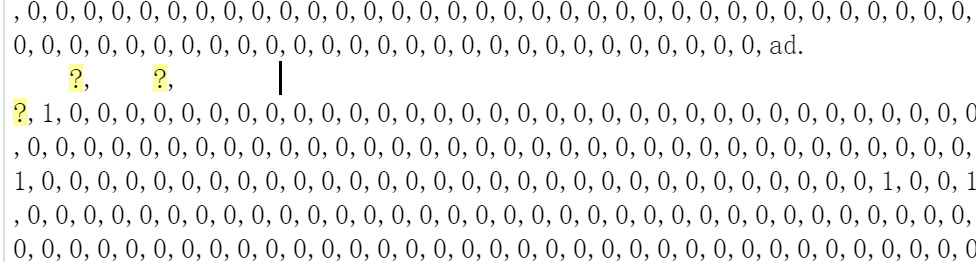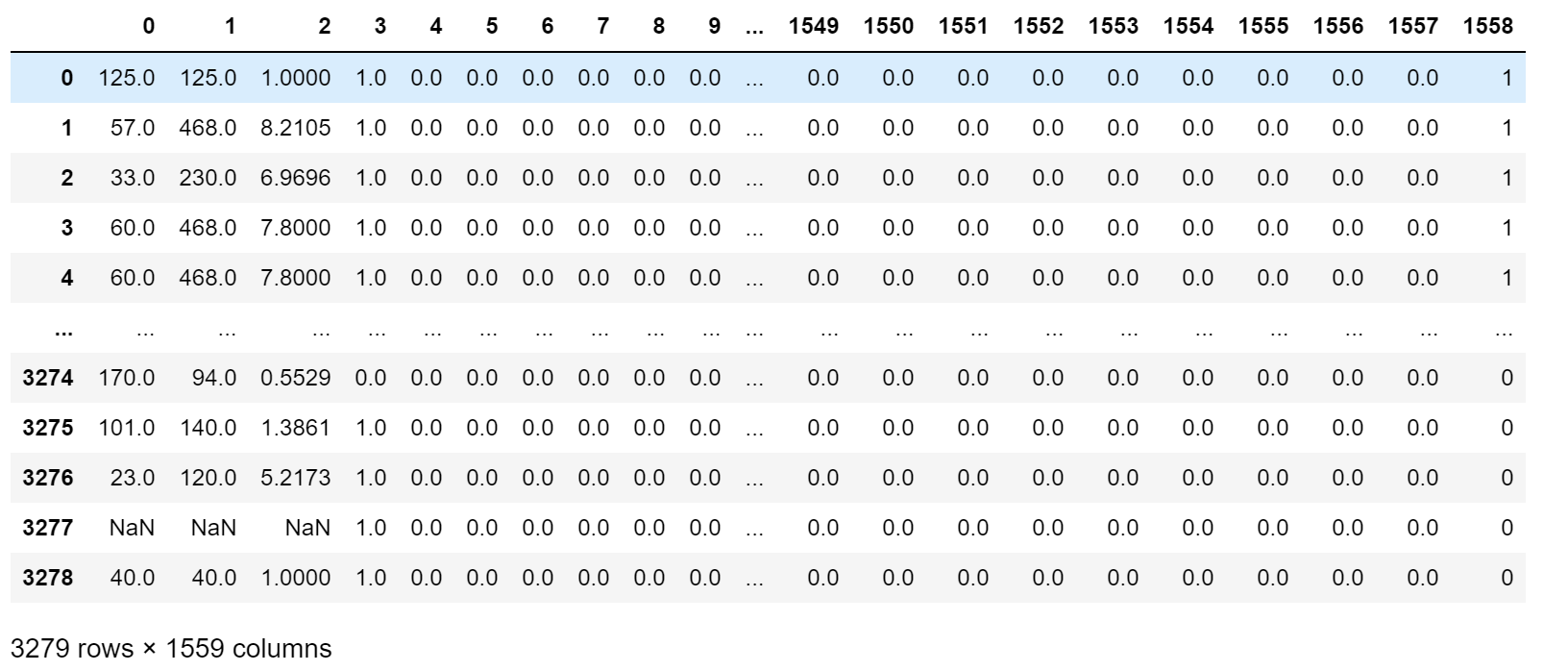data = ads_data.dropna(axis=0,how='any')
X = data.drop(1558,axis=1).values
Y = datafrom sklearn.tree import DecisionTreeClassifier
from sklearn.model_selection import cross_val_score
dtc = DecisionTreeClassifier(random_state=14)
score = cross_val_score(dtc, X, Y, scoring='accuracy')
print("准确度是：{}".format(score.mean()))from sklearn.decomposition import PCA
# n_components 表示的组成分的数量，默认返回数据集中所有的特征
pca = PCA(n_components=5)
Xd = pca.fit_transform(X)


pca.explained_variance_ratio_from sklearn.tree import DecisionTreeClassifier
from sklearn.model_selection import cross_val_score
dtc = DecisionTreeClassifier(random_state=14)
score = cross_val_score(dtc, Xd, Y, scoring='accuracy')
print("准确度是：{}".format(score.mean()))from matplotlib import pyplot as plt
from mpl_toolkits.mplot3d import Axes3D
Y = data
classes = set(Y)
colors = ['red', 'green']
fig = plt.figure()
ax = Axes3D(fig)

for cur_class, color in zip(classes, colors):

plt.legend()
plt.show()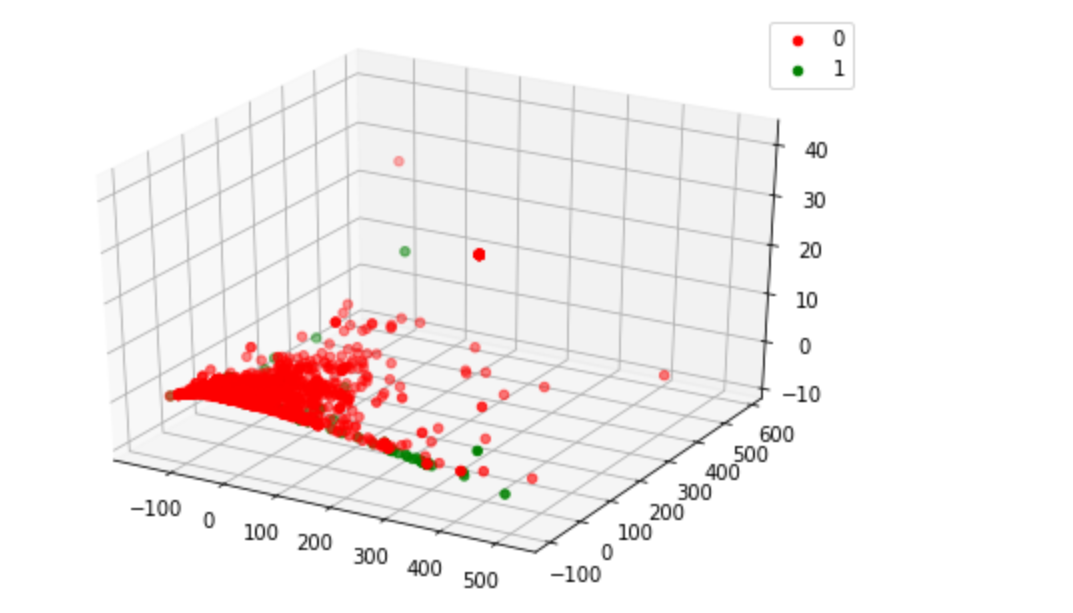• 方差
• 卡方验证
• 皮尔逊相关系数
• PCA算法

0
0 收藏

### 作者的其它热门文章0 评论
0 收藏
0